Hum tech electronics Electronics repair made easyAbout UsMission statementCRT Television troubleshooting guideCRT Television repair courseBasic electronics courseDVD Player repair guideCRT TV Repair Case Histories Vol.1CRT TV repair case histories Vol.2my blogRecommended booksContact UsSite Mapnewsletterfree ebook downloadUnderstanding markings on capacitor bodySONY TV DEAD repairedDo it yourself series lamp current limiter‘No disc” symptoms on JVC DVD player solvedWhat is the future of electronics repairing?Understanding how flyback transiformer worksUnderstanding markings on capacitor body
 Understanding markings on capacitor body. For electrolytic capacitors the marking are easy to understand and many technician have no problem here.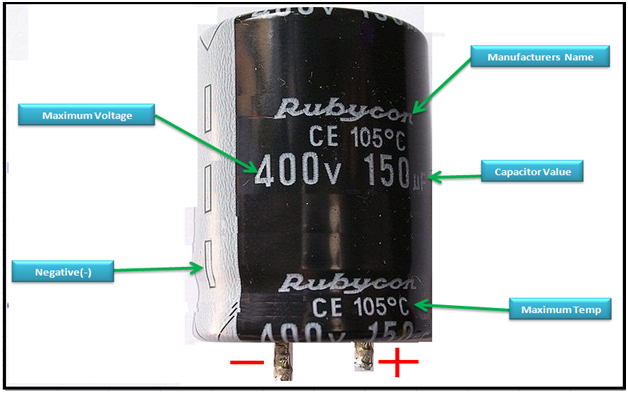The challenge is with non polar capacitor, let have a look at some of the marking on non polar capacitors. Let have look at a few examples: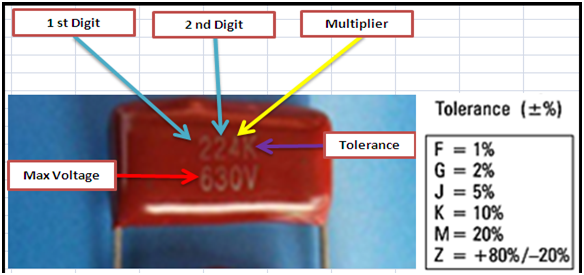Using the above method the result you get is always in pico farad(pF) Let us look at the above example of capacitor number 224K. Just write down the first and the second digit the way they are on the capacitor body. So we have 22 and then third digit is the number of zeros. Therefore 4 is equal to 4 zeros hence 224 is equal to 220000 pF. The result is always in pico farads(fF) And the K is the tolerance and from the table we can see K is equal to (+ or -) 10 % And finally the maximum voltage is 630 Volts. Let us look at another example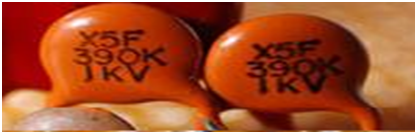So we have the first two digit 39 and add no zero, I mean you have 39 pF. If it was 391 then it could have been 39 then add one zero (0) and therefore could have been 39 0 pF again the tolerance is K therefore is +-10 % The maximum voltage is 1kV We know 1K=1000 units, therefore (1kV=1000V) Here is another example of non polarized capacitor written on the body 1n0K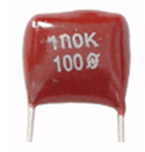This is the same as 1.0n capacitor: Given 1micro (uf) =1000nF 1nano=1000pf So 1.0n is equal to 1000pF and a tolerance of +-10% Maximum working voltage is 100Volts Tip: encoding ceramic capacitor body markings: White down the first two digits of the code, and then add the number of zeros that is indicated by the third digit of the code.   Examples: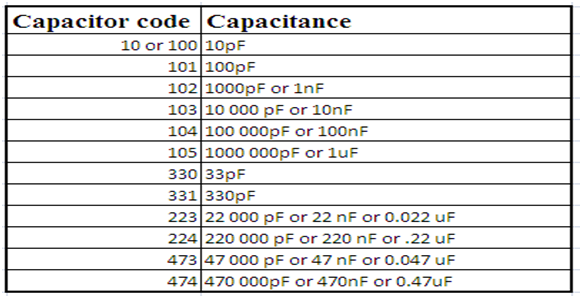Kindly note that capacitor 7n2=7k2=7200pF is one and the same thing.